Video Tutorial Calorimetry

Quick Notes Calorimetry

• Calorimetry is a technique used to find the heat energy change that occurs during a reaction.
• Heat energy change is found by measuring the temperature change of a substance that surrounds the reaction.
• Specific heat capacity (c) refers to the energy required to raise the temperature of 1g (or 1kg) of a substance by 1oC.
• The temperature change of a substance can be used with its specific heat capacity to find the energy it has absorbed or released.
• Q = mcΔT
• Change in energy = mass x specific heat capacity x change in temperature
• To increase the accuracy of calorimetry, heat loss must be minimised (extra insulation of a reactions surroundings can help).

Full Notes Calorimetry

In calorimetry, we measure the temperature change of a substance that surrounds a reaction. From this temperature change, we can calculate the energy change of the reaction.

To then find the enthalpy change for the reaction, we would need to divide the energy change by the number of moles reacting.

A common example of calorimetry is the combustion of a substance to heat a known volume (mass) of water.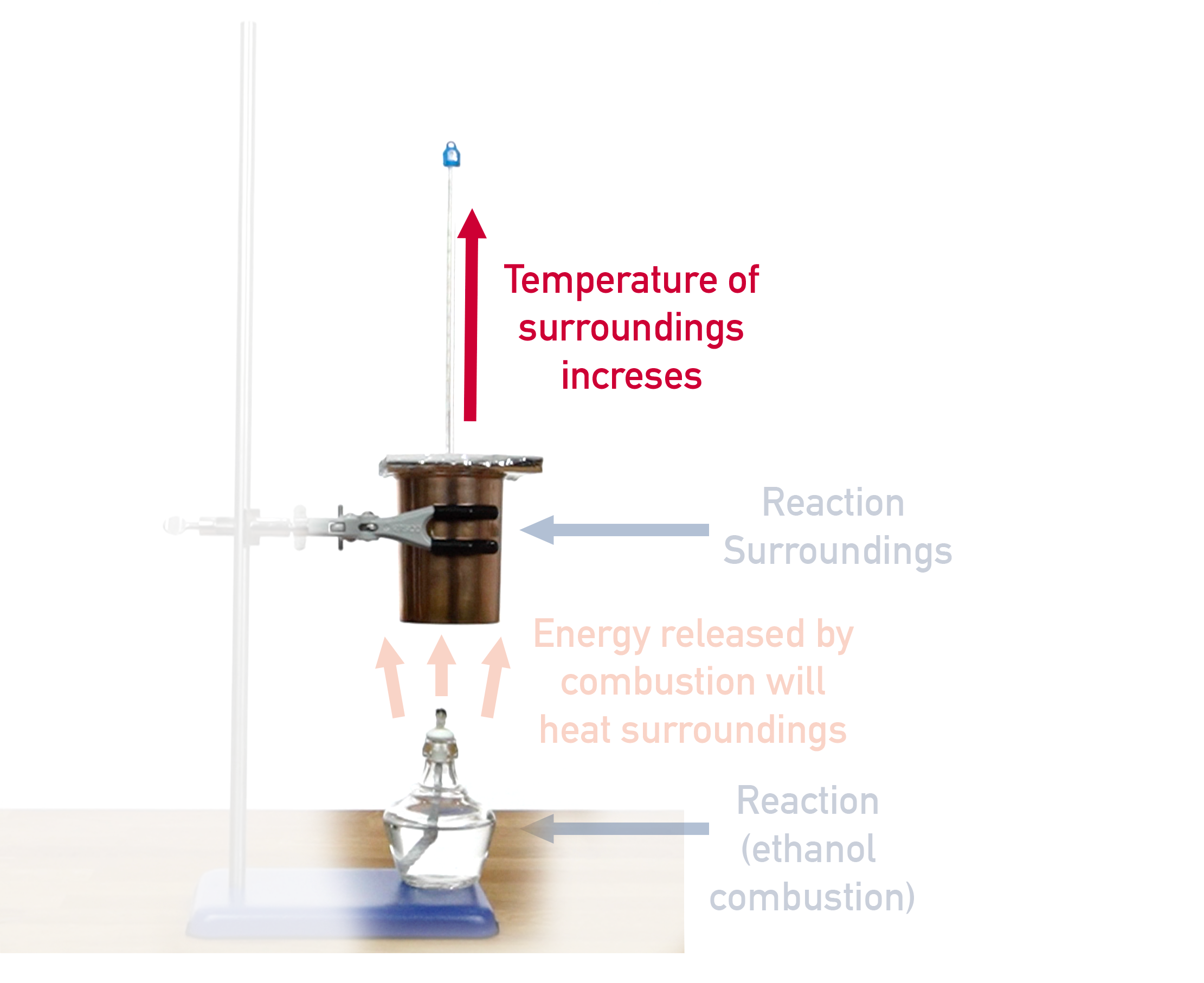Combustion reactions are exothermic, meaning heat is released from the combusting substance. The heat flows into the container of water held above the reaction. How much the temperature of the water changes is based on how much energy is released by the combustion reaction.

The container used to hold the water is called a calorimeter and is made of a highly conductive metal (such as copper) with thin walls. This means as much energy as possible flows into the water.

## Calculating Energy Change

To calculate how much energy has flowed into a reactions surroudnings we use the equation:

Q = mcΔT

Where:
Q = heat energy change
m = mass of surroundings
c = specific heat capacity of surroundings
ΔT = temperature change of surroundings

Different substances require different amounts of energy to heat up by a given temperature. For example, warming 10g of water by 10oC requires a different amount of energy to warming 10g of solid copper by 10oC.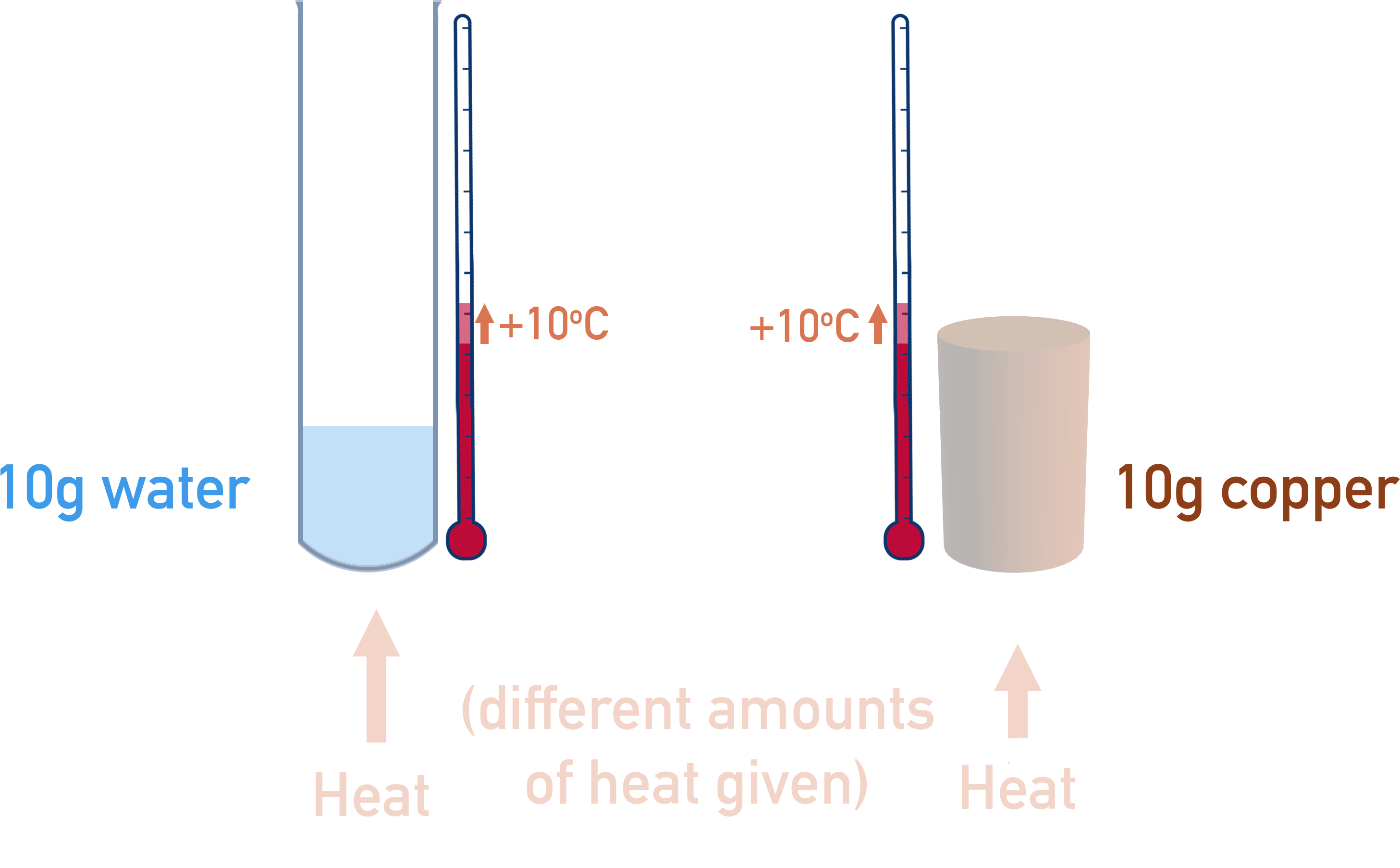To account for this, we have to use the specific heat capacity of the reactions surroundings. Specific heat capacity is the amount of energy it takes to heat 1g of a particular substance by 1oC. The units used are usually J g-1 K-1.

The mass is simply how much of the substance is being heated (or cooled) - it is not the mass of reactants! For water we can use 'mass = density x volume’ to find the mass of the water being used (density of water = 1g per cm3).

For Example...

An excess of solid magnesium is added to 100cm3 of Copper (II) Sulfate (CuSO4) solution that has a concentration of 1 mol dm-3.

Mg(s) + CuSO4(aq) → MgSO4(aq) + Cu(s)

The initial temperature of the solution was 25oC and the maximum temperature the solution reached was 60oC.

Find enthalpy change of the reaction.

Lets draw this out to see what is happening! The 'surroundings' of the reaction changing temperature is the water from the solution.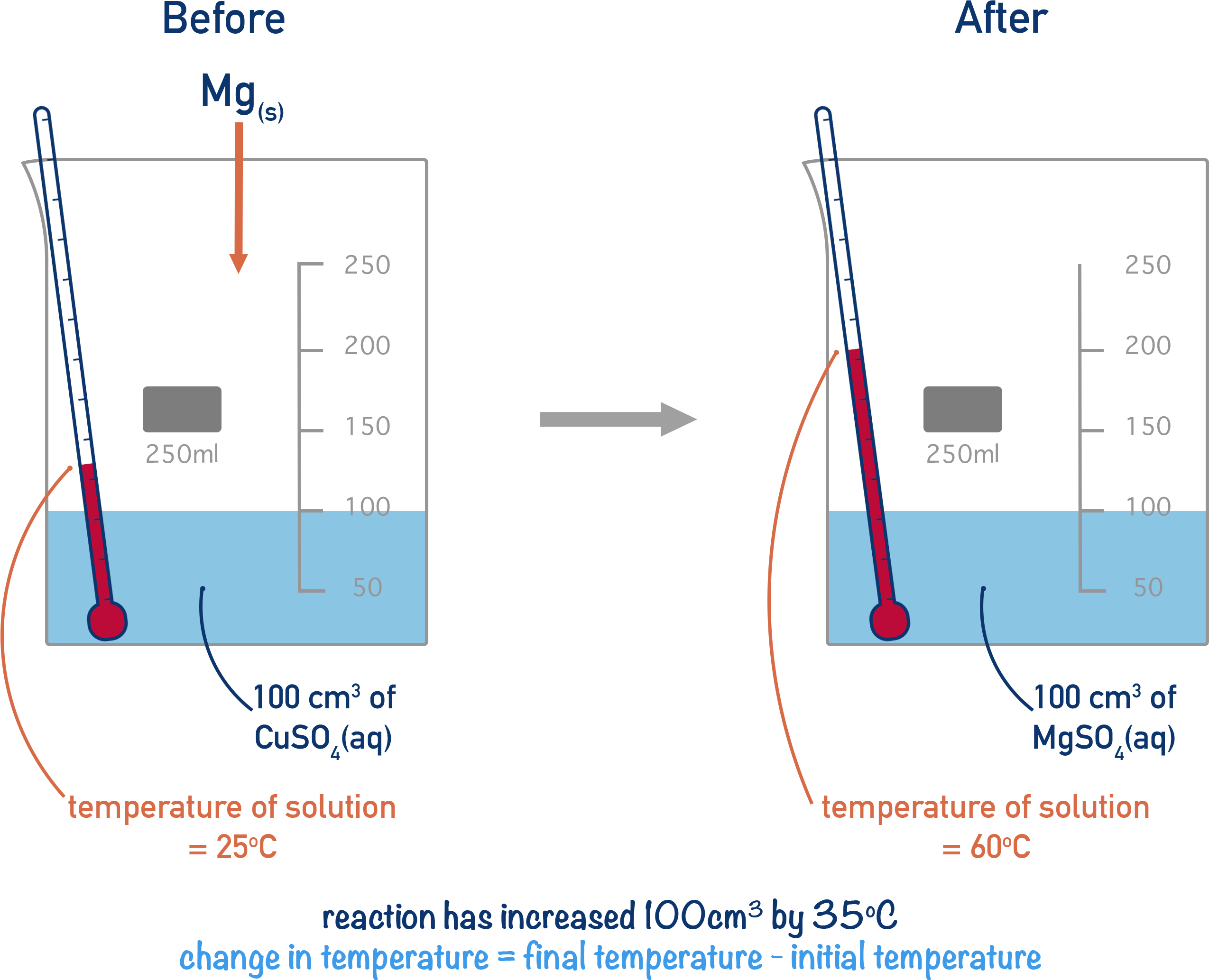First, we need to find the energy released by the reaction. To do this, we use the equation Q = mcΔT and substitute the values into the equation.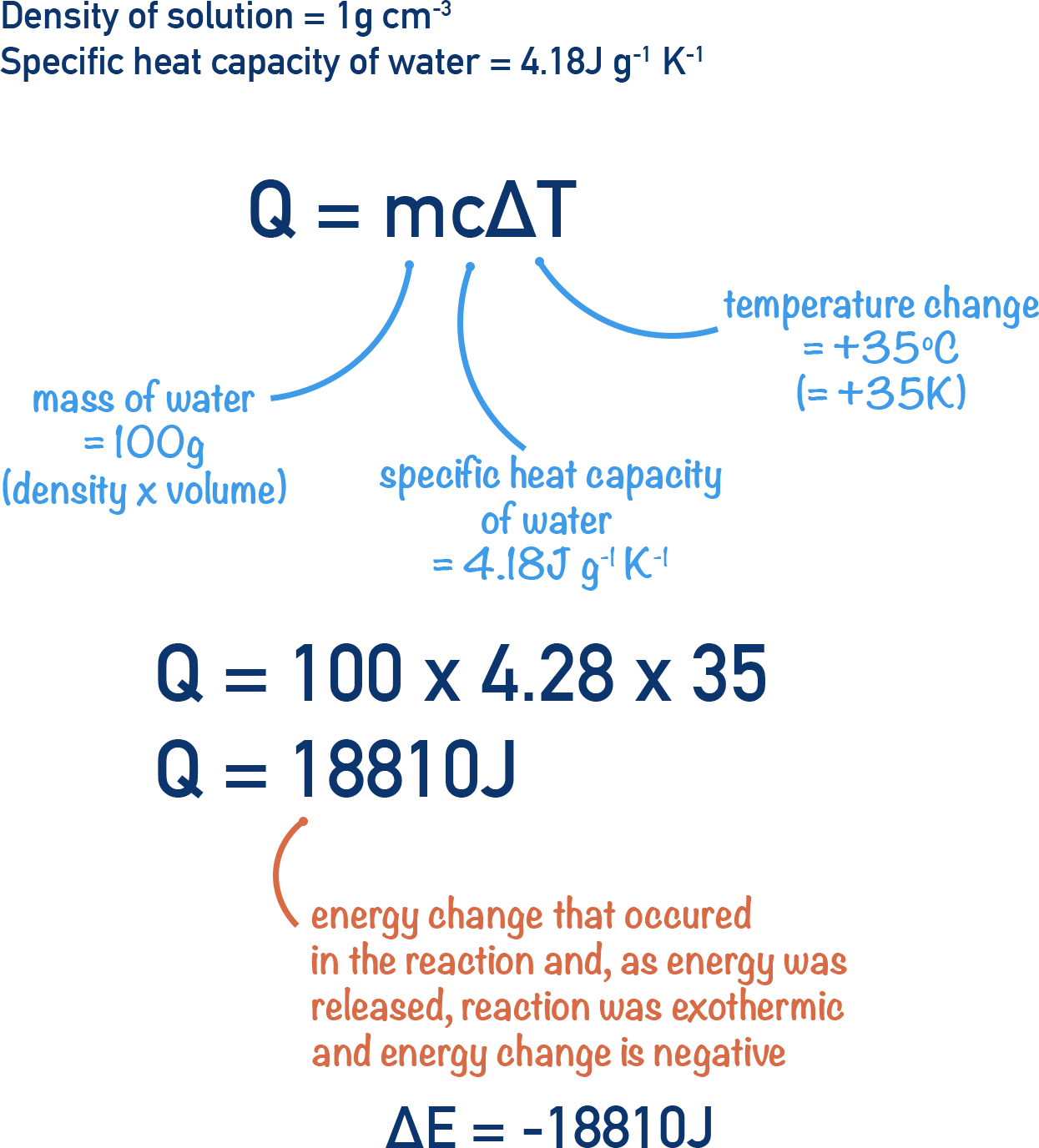The mass (m) of surroundings is taken to be 100g as the density of water is 1g per cm3. Note that the density of solution won't be exactly the same as water (see notes below).

Now we have the energy released in the reaction (-18810J) we need to find the enthalpy change, ΔH.

Enthalpy change is just the energy energy change per mol of reactant.

The equation for the reaction shows us that 1 mole of Mg reacts with 1 mole of CuSO4. The Mg is in excess meaning that it is the moles of CuSO4 that we are interested in.

The volume of solution used was 100cm3 and the concentration 1 mol dm-3.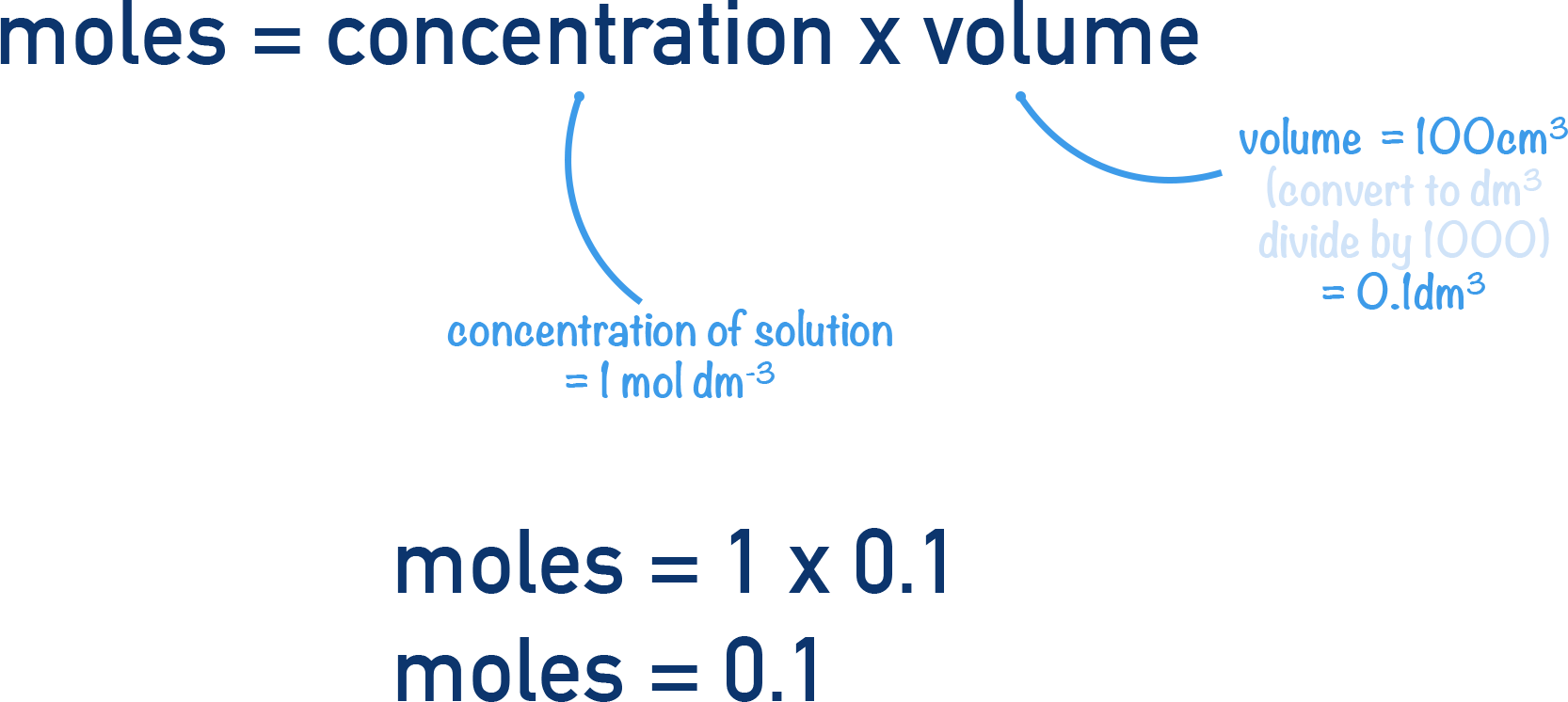This tells us that 0.1 moles of CuSO4 reacted and 18810 J of energy was released. To find how much energy would have been released per mol we just need to divide this energy value by the moles reacting.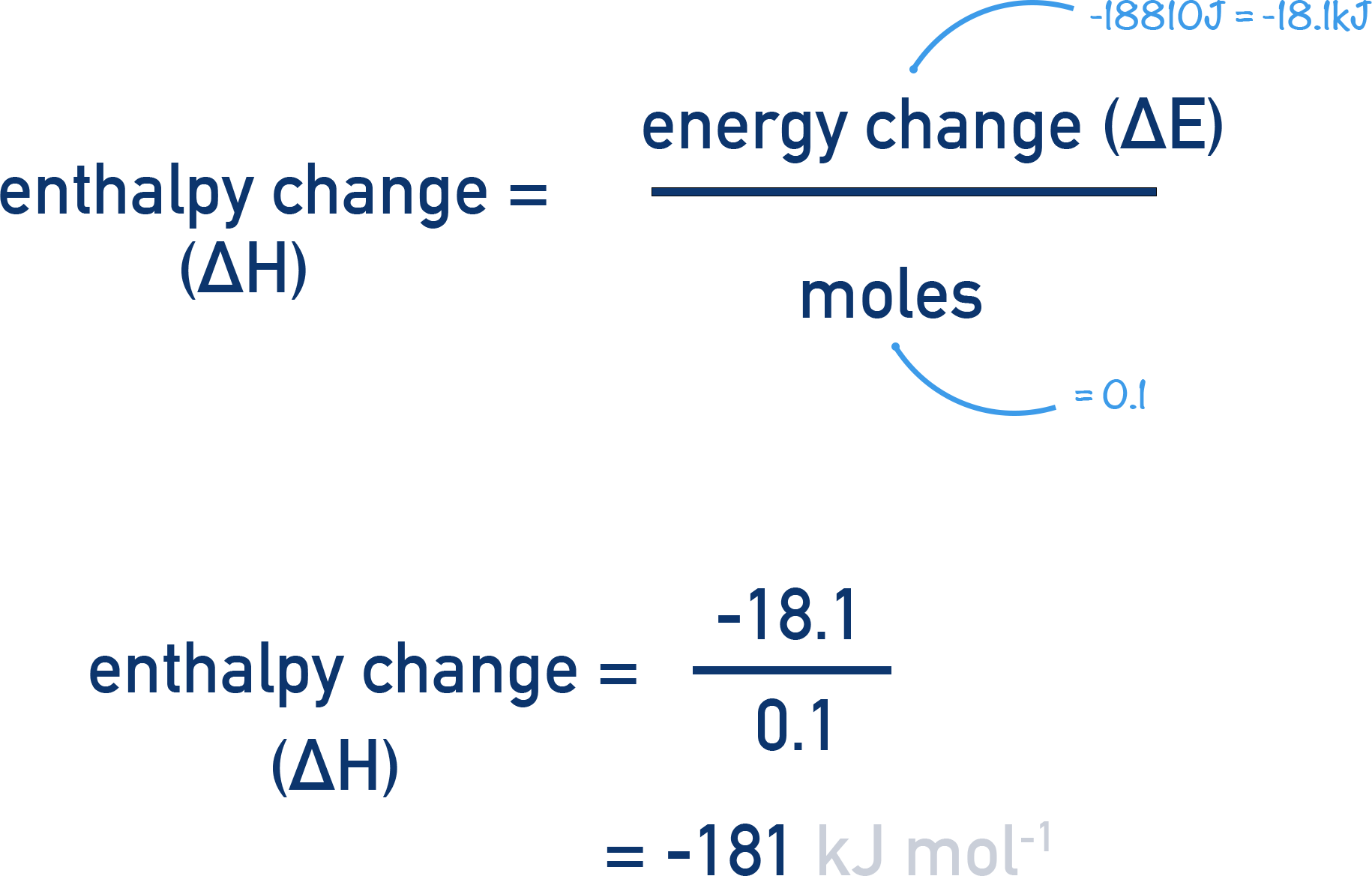## Potential Sources of Error

When dealing with solutions, water isn’t the only substance being heated, meaning the specific heat capacity of the solution won’t be exactly the same as for water. However, the difference between the value for water and for the solution is often negligable, so just the specific heat capacity for water is used. Be aware though, it’s a potential source of inaccuracy.

Note, the surroundings of a reaction are, technically, the entire universe!

For calorimetry, we assume the surroundings of the reaction to be a particular substance (for example, a certain volume of water). Obviously, as the temperature of this substance raises, it will also lose energy to its own surroundings – for example, the air or walls of its container. This is why calorimetry isn’t completely accurate without the use of advanced apparatus and, especially in classrooms(!), leads to lower energy change values than the actual energy change that occurs. This is due to heat loss from the substance, whose temperature is being measured, to the wider surroundings. Insulation can help minimise this heat loss.